This is the initialization value, meters.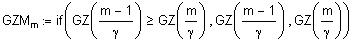The Maximum Available Righting Arm in this region is: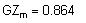Angle Corresponding to this Maximum Righting Arm, degrees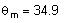Determine Limiting Angle, as per 46 CFR 173.095 (c)(2)
Limiting Angle, the least of 40 degrees, Angle of Maximum Righting Arm and Downflooding Angle.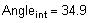Sheet 2 of 3
Determine Intercept (or Equilibrium) Angle Between Heeling Arm Curve & Righting Arm Curve, as per 46 CFR 173.095 (c)(1) and (2)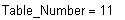Initial Angle of Range needed for iterations below, degrees.
A cubic spline is used to represent the end conditions.The above function is redefined below in terms of a cubic interpolation function.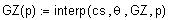This function is graphed on the last page of this analysis.
Calculations for Heeling Arm Curve, as per Part 46 CFR 173.095 (d)
In this section the Heeling Arm Curve is calculated through formula at right.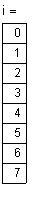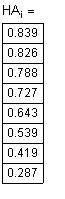The formula for representing HA (heeling arm) as a function of theda (heel angle) is derived as follows:
A cubic spline is used to represent the end conditions.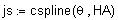The above function is redefined below in terms of a cubic interpolation function.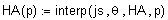This function is also graphed on the last page of this analysis.
Determine Angle of Maximum Righting Arm, as per 173.095(c)(2)(i)
Determining Maximum Righting Arm and its Corresponding Angle
The Angle corresponding to maximum righting arm is obtained from the following iterative process.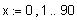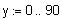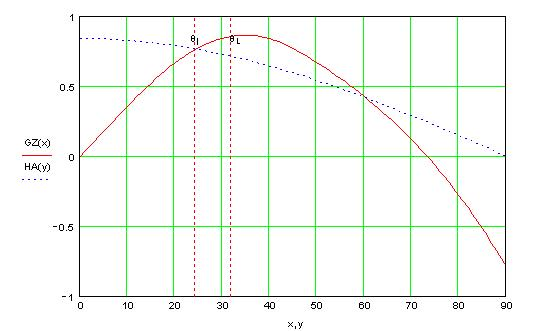Summary of Important Inputs
Summary of Calculated Results
Downflooding Angle, degrees
Intercept Angle, degrees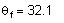Residual Area Required Under RA Curve, meters·deg
Residual Area Available Under RA Curve, meters·deg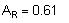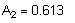Draft, meters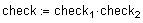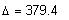Displacement, metric tons
Results of this analysis, satisfactory only if equal to one.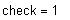Vertical Center of Gravity, metersSheet 3 of 3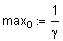Iteration Fineness, fractions of a degree
Iteration Values, first value of range important because of multiple intercepts possible for some cases, degrees.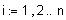Tolerance Allowed on GZ Difference, meters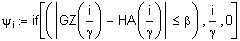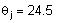Intercept Angle
Check Equilibrium Angle to see if Less than Downflooding Angle, per 46 CFR 173.095 (c)(1)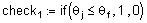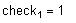Check Energy to Limiting Angle, as per 46 CFR 173.095 (c)(2)
Calculate Area from Intercept Angle up to Limiting Angle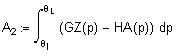Check if meets Minimum Residual Moment Area requirementsGraph of Righting Arm Curve
or Curve of Statical Stability
*
26.5m x 9.5m x 4.3m
*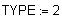Filename of the calculations that are contained here.
*
TBL-F1B.MCD
Vertical Center of Gravity, meters, above Baseline. The is the same VCG (or KG) value that was used for constructing the "Cross Curves of Stability." This value facilitates fast cross checking with the other vessel data.
Vessel Draft, meters. When INPUT =1 put in the mean draft for the condition under evaluation. When INPUT=2 it usually means the level draft is being evaluated. Otherwise it is the LCF draft.
*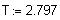*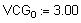Downflooding Angle, based on the above draft and the vessel's geometry. Part 46 CFR 173.095 (e) requires the location where the hull does not close automatically.
*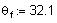Condition Inputs from Hydrostatics Program or Curves of Form & Cross Curves of Stability
Input Righting Arms are below. No free surface effects are included in this data .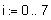*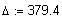Vessel Displacement, Metric Tons.
Keel to Metacenter for the Transverse Direction, meters, this value obtained at the same time as the above displacement for the level trim case. Where KMT = VCB + BMT.
Towline Pull, Dynamic Criterion, VCG Check, as per 46 CFR 173.095(c) - (f)
*
Table F1B
*
Date: 13 July 2004
This analysis is based on the dynamic requirements contained in 46 CFR 173.095 (c) through (f). These requirements are an option to the static requirements stated by 46 CFR 173.095 (b). This analysis was prepared in a Windows 95 operating system (or later version) with MathCAD version 6 (or later version).
*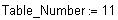*
Units: Metric
Vertical Center of Gravity, meters, above the Baseline is positive. This value should already include the free surface correction.
General Inputs for Vessel
**
Tug Company Name
Client
*
M/T Tugboat Name
Vessel Name
TYPE =1 when a specific VCG is being checked. TYPE=2 when seeking the maximum allowable value for this criterion. In a TYPE 2 analysis this VCG value is ITERATED to obtain the highest possible value that meets all this criterion's requirements.
*
Z-Drive Tug
Vessel Type
Vessel Particulars,
LBP x Beam x Depth
*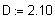Propeller Diameter, meters
Minimum Residual Moment Area Required, in metric units of meter·degrees
*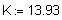Formula Constant for Metric units
*Metacentric Height Calculation
Sheet 1 of 3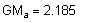Available Metacentric Height, metersCalculations for Righting Arm Curve
In this formula a correction for VCG0 is made to the Righting Arm Curve.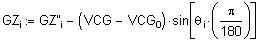The last column of this table shows Corrected Righting Arms for VCG
The formula for representing GZ (righting arm) as a function of theda (heel angle) is derived as follows: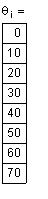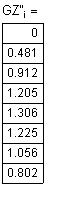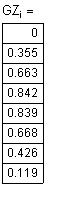*
*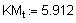*Longitudinal Center of Gravity, meters, aft of Midships is positive. For the level trim case this equals the LCB value and it is obtained at the same time as the displacement above.
*If Input = 1 data for this condition is directly from a hydrostatics program, if Input =2 data are from the "Curves of Form" and the "Cross Curves of Stability."
*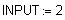Vessel Inputs for Towing as Defined by 46 CFR 173.095 (b)
fraction applicable to Z-Drive Tugs, value from Static Requirements.
*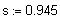*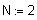Number of Propellers
*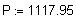Shaft Power, per shaft, KW
Maximum Ve rtical Distance from propeller shaft centerline to towing bitts, meters
*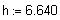The procedures, techniques and presentation contained on these pages are copyrighted. Only purchasers of the template may utilize them, any other use is strictly prohibited.